ICSE Class 9 Maths Sample Question Paper 1 with Answers

Max Marks :80
[2 Hours]

General Instructions

• Answers to this Paper must be written on the paper provided separately.
• You will not be allowed to write during the first 15 minutes.
• This time is to be spent in reading the question paper.
• The time given at the head of this Paper is the time allowed for writing the answers.
• Section A is compulsory. Attempt any four questions from Section B.
• The intended marks for questions or parts of questions are given in brackets [ ].

Section – A [40-Marks]
(Attempt all questions from this Section)

Question 1.
(a) Rationalize the denominator : $$\frac{14}{5 \sqrt{3}-\sqrt{5}}$$(b) Factorize the given expression completely : 6×2 + 7x – 5 
6 x2+ 7x-5 = 6x2 + (10 – 3)* – 5
– 6x2 + 10x- 3x – 5
= 2x(3x + 5) – 1(3x + 5)
= (3x + 5) (2x – 1).(c) In the given figure, AB = $$\frac{1}{2}$$ BC, where BC = 14 cm. Find : 
(ii) Area of ΔABC
(iii) Area of semicircle
Hence find the area of shaded region. Use 7π = $$\left(\text { Use } \pi=\frac{22}{7}\right)$$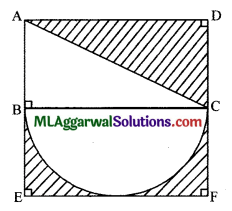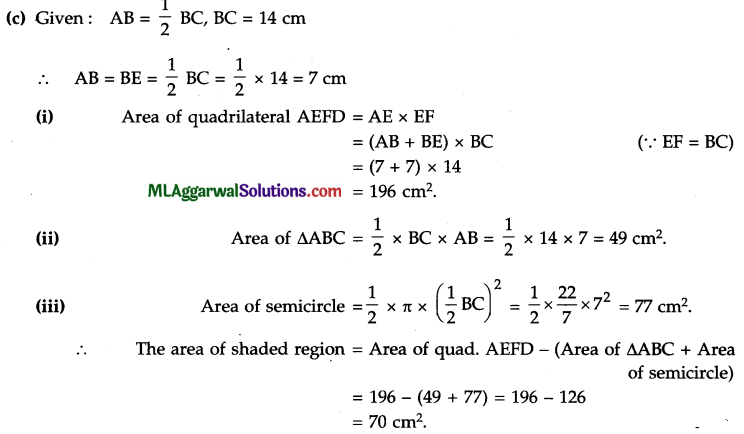Question 2.
(a) Mr. Ravi borrows ₹ 16,000 for 2 years. The rate of interest for the two successive years are 10% and 12% respectively. If he repays ₹ 5,600 at the end of first year, find the amount outstanding at the end of the second year. 

(b) Simplify: $$\left(\frac{8}{27}\right)^{-\frac{1}{3}} \times\left(\frac{25}{4}\right)^{\frac{1}{2}} \times\left(\frac{4}{9}\right)^{0}+\left(\frac{125}{64}\right)^{\frac{1}{3}}$$ 

(c) In the given figure, ABCD is a parallelogram. AB is produced to P, such that AB = BP and PQ is drawn parallel to BC to meet AC produced at Q.
Given AB = 8 cm, AD = 5 cm, AC = 10 cm,
(i) Prove that point C is mid-point of AQ.
(ii) Find the perimeter of quadrilateral BCQP.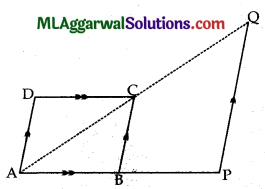(a) Here, P = ₹ 16000
For first year: R = 10% , T = 1 year
∴ $$\text { Interest }=\frac{16000 \times 10 \times 1}{100}= 1600$$
Amount = ₹ (16000 + 1600) = ₹ 17600
∴ Amount repaid = ₹ 5600.For Second Year :
P = (17600 – 5600) = 12000, R = 12% , T = 1 year
∴ Intrest = $$\frac{12000 \times 12 \times 1}{100}$$ = ₹1440
∴ Amount =(12000+1440) = ₹13440

(b)(c) Given : ABCD is parallelogram, AB = BP, AB = 8 cm, AD = 5 cm, AC = 10 cm.
(i) ∵ AB = BP
∴ B is mid-point of AP
Also, BC || PQ (Given)
AC = CQ (By mid-point theorem)
∴ C is mid-point of AQ. Hence Proved.

(ii) BP = AB = 8 cm (Given)
BC = AD = 5 cm (∵ ABCD is a parallelogram)
CQ = AC = 10 cm [From part, (i)]
PQ = 2 BC = 2×5 = 10 cm(By mid-point theorem)
∴ Perimeter of quadralateral BCQP = BP + PQ + CQ + BCQuestion 3.
(a) Solve following pairs of linear equations using cross-multiplication method : 
5x – 3y = 2
4x + 7y = – 3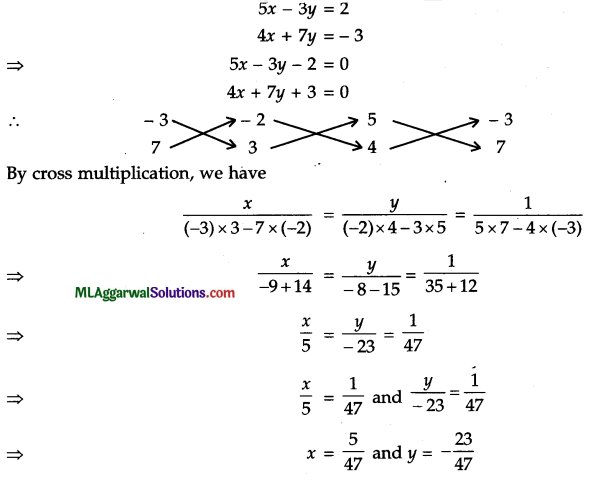(b) Without using tables, evaluate : 
$$4 \tan 60^{\circ} \sec 30^{\circ}+\frac{\sin 31^{\circ} \sec 59^{\circ}+\cot 59^{\circ} \cot 31^{\circ}}{8 \sin ^{2} 30^{\circ}-\tan ^{2} 45^{\circ}}$$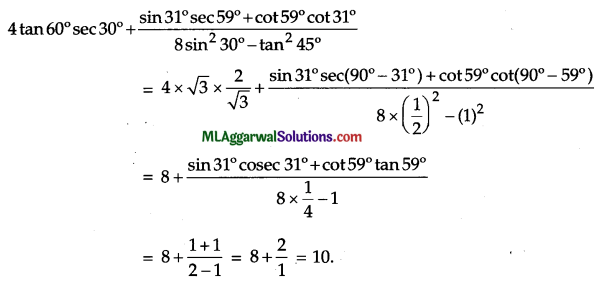(c) Construct a frequency polygon for the following frequency distribution, using a graph sheet. 

 Marks 40-50 50-60 60-70 70-80 80-90 90-100 No. of students 7 18 26 37 20 6

Use : 1 cm = 10 marks, 1 cm = 5 students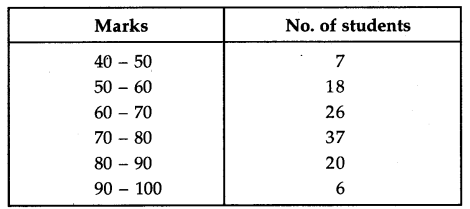Question 4.
(a) Evaluate : 3 log 2 – $$\frac{1}{3}log 27 + log 12 – log 4 + 3 log 5$$.(b) If x –$$\frac{1}{x}$$ =3, evaluate x3 – $$\frac{1}{x^{3}}$$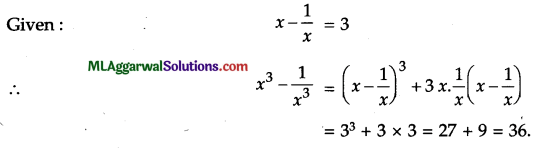(c) In the given diagram, O is the centre of the circle and AB is parallel to CD. AB = 24 cm
and distance between the chords AB and CD is 17 cm. If the radius of the circle is 13 cm, find the length of the chord CD.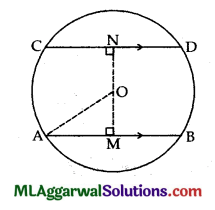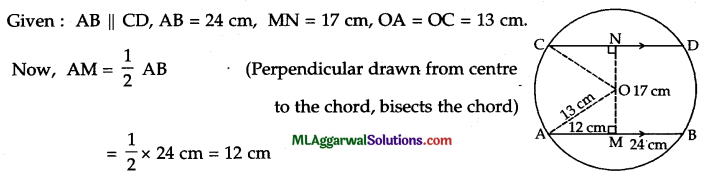Section – B [40 Marks]
(Attempt any four questions from this Section)

Question 5.
(a) Find the coordinates of the points on Y-axis which are at a distance of 5√2 units from
the point (5, 8). 
(a) Let the coordinates of the point on Y-axis be (0, y).
Distance = 5 √2
⇒$$\sqrt{(0-5)^{2}+(y-8)^{2}}=5 \sqrt{2}$$
Squaring both sides, we get
(0-5)2 + (y-8)2 = (5-√2)2
⇒ 25 + y2 – 2 y-8 + 64 = 50
⇒ y2 – 16y + 89 – 50 = 0
⇒ y2 – 16y + 39 = 0
⇒ y2 – (13 + 3)y + 39 = 0
⇒ y2-13y-3y+ 39 =0
⇒ y(y – 13) – 3(y – 13) = 0
⇒ (y – 13) (y – 3) = 0
⇒ y-13=0 or y-3 = 0
⇒ y = 13 or y = 3.
.’. The required point is (0,13) or (0, 3).

(b) In the given figure, BC is parallel to DE. Prove that area of ΔABE = Area of ΔACD.Given: BC || DE
∴ Area of ΔBCE = Area of ΔBCD
(Triangles, on same base and between the same parallels are equal in area)
⇒ Area of ΔBCE + Area of ΔABC = Area of ΔBCD + Area of ΔABC
(Adding area of ΔABC to both sides) .
⇒ Area of ΔABE = Area of ΔACD. Hence Proved.

(c) A stun of ₹ 12,500 is deposited for 1 $$\frac{1}{2}$$ years, compounded half-yearly. It amounts to ₹ 13,000 at the end of first half year. Find : 
(i) The rate of interest
(ii) The final amount. Give your answer correct to the nearest rupee.
P = ₹ 12,500, A = ₹ 13,000, T = – year.
∴ Interest for $$\frac{1}{2}$$ year
= ₹ (13000 – 12500) = ₹ 500.
(i) Let R be the rate of interest.
∴ $$\mathrm{R}=\frac{\mathrm{I} \times 100}{\mathrm{P} \times \mathrm{T}}=\frac{500 \times 100}{12500 \times \frac{1}{2}}=8$$
∴ The rate of interest = 8 % p.a.(ii) Now, n = 1 $$\frac{1}{2}$$years =$$\frac{3}{2}$$years.
C.I. is calculated half-yearly,
$$\mathrm{R}=\frac{\mathrm{I} \times 100}{\mathrm{P} \times \mathrm{T}}=\frac{500 \times 100}{12500 \times \frac{1}{2}}=8$$

Question 6.
(a) Construct a parallelogram ABCD in which AB = 6.4 cm, AD = 5.2 cm and the
perpendicular distance between AB and DC is 4 cm. 
(a) Given : AB = 6.4 cm, AD = 5.2 cm,
Perpendicular distance between AB and DC is 4 cm.Steps of construction :
(1) Draw a line segment XY and take any point P on it.
(2) At P, draw a perpendicular PZ and cut-off PD = 4 cm.
(3) From D, cut-off XY at A such that DA = 5.2 cm.
(4) From A, cut-off XY at B such that AB = 6.4 cm.
(5) From B and D, draw arcs of 5,2 cm and 6.4 cm radii respectively which intersect at C.
(6) Join AD, BC and CD to obtain the required parallelogram ABCD.

(b) Factorize : 4a2 – 9b2 – 16c2 + 24be 
4a2 – 9b2 – 16c2 + 24 be =4a2– (9b2 – 14bc + 16c2)
= (2a)2 – {(3b)2 – 2-3b-4c + (4c)2}
= (2a)2 – (3b – 4c)2
= (2a + 3b – 4c) (2a – 3b + 4c).(c) In the given diagram, ABCD is a parallelogram, ΔAPD and ΔBQC are equilateral triangles.
Prove that: . 
(i) ∠PAB = ∠QCD
(ii) PB = QD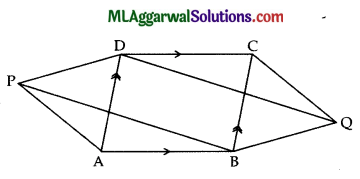Given : ABCD is parallelogram, ΔAPD and ΔBQC are equilateral triangles.
(i) ∠DAB = ZBCD (Opp. angles of a || gm are equal)
⇒ ∠DAB + ∠PAD = ∠BCD + ∠BCQ (∠PAD = ∠BCQ = 60°)
⇒ ∠PAB = ∠DCQ. Hence Proved.

(ii) In ΔPAB and ΔQCD,
AB DC (Opp. sides of aIgm are equal)
∠PAB = ∠QCD [From (i)
∠PAB ≅ ΔQCD (SAS axiom)
PB = QD (c.p.c.t.)
Hence Proved.Question 7.
(a) Solve for x : sin2 x + cos2 30° = $$\frac{5}{4}$$; where 0° ≤ x ≤ 90°(b) Evaluate for x :$$\left(\sqrt{\frac{5}{3}}\right)^{x-8}=\left(\frac{27}{125}\right)^{2 x-3}$$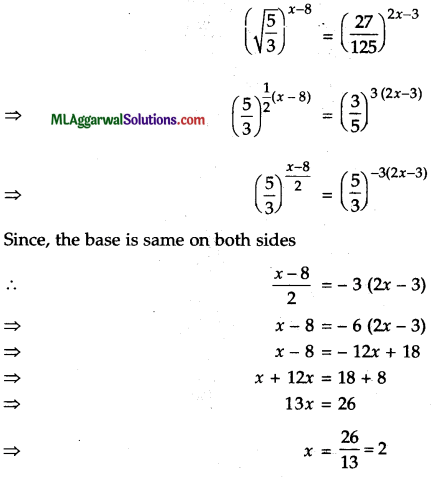(c) In the given figure, triangle ABC is a right angle triangle with ∠B = 90° and D is mid­point of side BC. Prove that AC2 = AD2 + 3 CD2.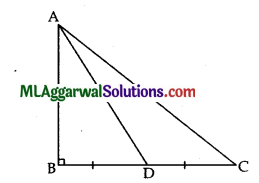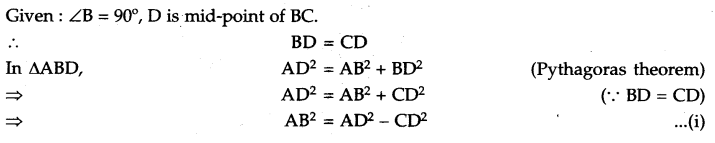Question 8.
(a) In the given figure, ∠ABC = 66°, ∠DAC = 38°. CE is perpendicular to AB and AD is perpendicular to BC. Prove that CP > AP.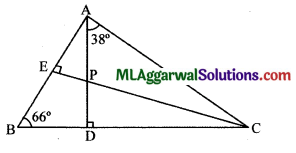Given: ∠ABC = 66°, ∠DAC = 38°, CE ⊥AB, AD ⊥ BC.
In ∠ABD, ∠BAD + ∠ABD = ∠ADC (Exterior angle is equal to sum
of interior opposite angles)
In ∠SACE, ∠ACE + ∠AEC + ∠CAE = 180° (Sum of angles in a triangle is 180°)
∠ACE + 90° + (24° + 38°) = 180°
∠ACE + 152° = 180°
∠ACE = 180° – 152° = 28°.
Now, ∠CAP > ∠ACP ( 38°> 28°)
CP > AP (In a triangle, greater angle has greater side opposite to it)
Hence Proved.

(b) Mr. Mohan has ₹ 256 in the form of ₹ 1 and ₹ 2 coins. If the number of ₹ 2 coins are three more than twice the number of ₹ 1 coins, find the total value of ₹ 2 coins. 
Total amount = ₹ 256
Let the no. of ₹ 1 coins be x and that of ₹ 2 coins be y.
∴ Value of x coins = ₹ 1 × x = ₹  x
Value of y coins = ₹ 2 x y = ₹ 2y.
∴ x + 2y = 256
Also, y = 3 + 2x
Using equation (ii) in (i), we have
Also, y=3+2x
Using equation (ii) in (i), we have
⇒ x+2(3+2x)= 256
⇒ x+6+4x= 256
⇒ 5x =256 – 6
⇒ x=$$\frac{250}{5}$$=50.
Putting the value of x in equation (ii), we get
y =3+2x 50 =3+ 100 = 103.
∴ Total value of ₹ 2 coins = ₹ 2y
=₹ 2x 103
=₹ 206.(c) Find (i) mean and (ii) median for the following observations : 
10, 47, 3, 9, 17, 27, 4, 48, 12, 15
Given observations are 10, 47, 3, 9, 17, 27, 4, 48, 12, 15.
Here, n 10
(i) Σx = 192
$$\text { Mean }=\frac{\Sigma x}{n}=\frac{192}{10}=19.2$$

(ii) Rearranging the observations in ascending order, we have
3, 4, 9, 10, 12, 15, 17, 27, 47, 48Question 9.
(a) Three cubes are kept adjacently, edge to edge. If the edge of each cube is 7 cm, find total surface area of the resulting cuboid. 
Given : Length of each side of cube = 7 cm
For cuboid, 7cm
l= (7 + 7 + 7) cm = 21 cm
b = 7 cm, h = 7 cm.
We know, Total surface area = 2 (lb + bh + Ih)
= 2 (21 x 7 + 7 x 7 + 21 x 7)
= 2 (147+ 49 + 147) = 2 x 343 = 686 cm2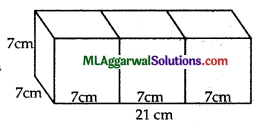(b) In the given figure, arc AB = twice (arc BC) and ∠AOB = 80°. Find : 
(i) ∠BOC
(ii) ∠OAC(i) Given: Arc AB = 2 (arc BC),∠AOB =80°
∠AOB=2∠BOC
∠BOC = $$\frac{1}{2}$$ ∠AOB
$$\frac{1}{2}$$ × 80°
=40°

(ii) In ΔAOC
⇒ ∠OCA = ∠OAC (Angles opposite to equal
sides are equal)
Now, ∠OAC + ∠AOC + ∠OCA = 180° (Angle sum property)
∠OAC + (∠AOB + ∠BOC) + ∠OAC = 180° (∠OAC= ∠OCA)
= 2∠OAC + (80° + 40°) = 180°
2∠OAC + 120° = 180°
2∠OAC = 180° – 120° = 60°
∴ ∠OAC =$$\frac{60^{\circ}}{2}$$ 3o°

(c) Solve graphically the following system of linear equations (use graph sheet): 
x – 3y = 3
2x + 3y = 6
Also, find the area of the triangle formed by these two lines and the Y-axis.
x – 3y = 3 …………….. (i)
2x + 3y = 6 ………. (ii)
from equation (i)
x = 3y + 3

 X 3 0 -3 y 0 -1 -2

The points are (3, 0), (0, – 1), (- 3, – 2).
From equation (ii),
⇒ 2x = 6 – 3y
⇒ $$x=\frac{6-3 y}{2}$$X 3 0 -3 y 0 2 4

The points are (3, 0), (0, 2), (- 3, 4).
These points are plotted on the graph.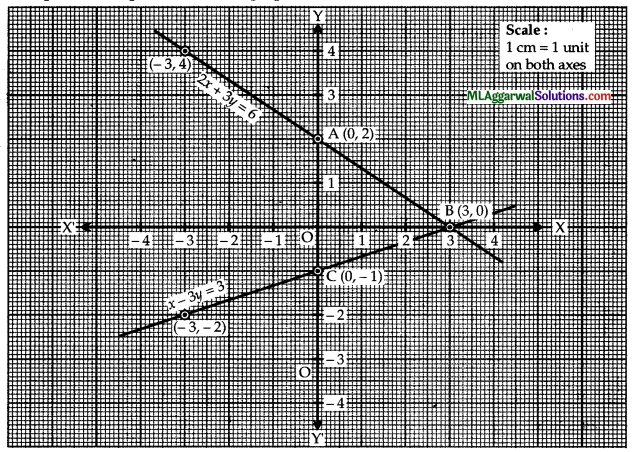The two lines intersect at the point (3, 0).
∴ x=3,y=0
Triangle formed by the lines (i), (ii) and Y-axis is ABC.Question 10.
(a) Each interior angle of a regular polygon is 135°. Find : 
(i) The measure of each exterior angle.
(ii) Number of sides of the polygon.
(iii) Name the polygon.
(a) Given: Each interior angle = 135°
(i) Exterior angle = 180° – 135° = 45°(iii) The polygon is a regular octagon.

(b) If log 4 = 0.6020, find the value of log 80. 
Given : log 4 = 0.6020
⇒ log 22 = 0.6020
⇒ 2 log 2 = 0.6020
⇒ log 2
$$=\frac{0.6020}{2}$$
Now, log 80 = log (8 x 10) = log 8 + log 10
= log 23 + log 10 = 3 log 2 + log 10
= 3 x 0.3010 + 1 = 1.9030(c) Evaluate x and y from the figure diagram.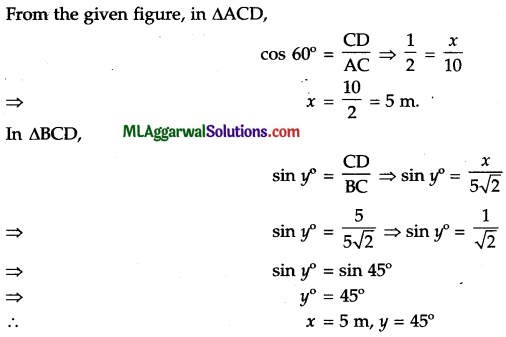Question 11.
(a) ΔABC is an isosceles triangle such that AB = AC. D is a point on side AB such that
BC = CD. Given ∠BAC = 28°. Find the value of ∠DCA. 
(b) Prove that opposite angles of a parallelogram are equal. 
(c) The cross-section of a 6 m long piece of metal is shown in the figure. Calculate : 
(i) The area of the cross-section
(ii) The volume of the piece of metal in cubic centimetres.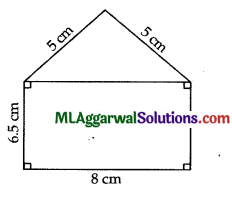(a) Given : AB = AC, BC = CD, ∠BAC = 28°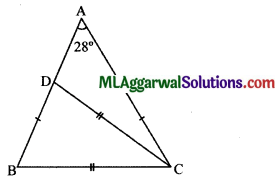Since, AB = AC
∠ABC = ∠ACB. (Equal sides have equal angles opposite to them)
∠ABC + ∠ACB + ∠BAC = 1800 (Sum of angleš in a triangle is 1800)
∠ABC + ∠ABC + 28° = 180°
2∠ABC =180°-28°
∠ABC= $$\frac{152^{\circ}}{2}$$=76°
∠BDC = ∠CBD = 76°
Now, ∠ACD + ∠CAD = ∠BDC (Exterior angle is equal to sum of interior opposite angles)
∠ACD + 28° = 76°
∠ACD = 76° – 28° = 480

(b) Given : A parallelogram ABCD.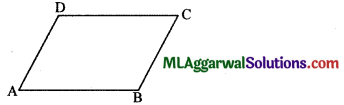To prove:∠A = ∠C and ∠B = ∠D.
Proof: AB II DC, AD II BC ( ABCD is a parallelogram)
∠A + ∠D = 1800 (Co-interior angles) …(i)
and ∠D + ∠C = 180° (Co-interior angles) …(ii)
From (i) and (ii),∠A + ∠D =∠D + ∠C
∠A=∠C
Similarly,∠B = ∠D.Hence Proved.

(c) In triangle, length of equal sides (a) = 5 cm, base (b) = 8 cm.
In rectangle, Length (L) = 8 cm, Breadth (B) = 6.5 cm.
(i) The area of cross-section = Area of rectangle + Area of triangle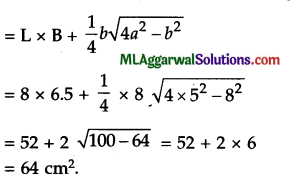(ii)  Length of metal = 6 m = 600 cm.
Volume = Area of cross-section x Length
= 64 cm2 x 600 cm
= 38400 cm3.